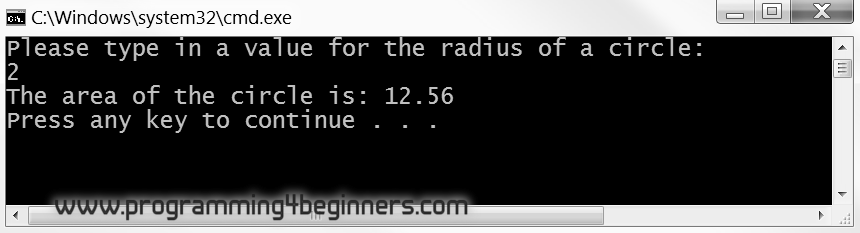## 4. Variables

### Execution by Hand

As you have seen, a variable can be understood as a name given to a value. For this association to work, there must be some mechanism for storing values of variables, so that a stored value can be retrieved whenever it is needed. This mechanism is known as memory. During the process of executing a program by hand, this memory will be nothing more than a piece of paper set aside for writing down all the names together with values that have been assigned to them. To give you one example of how you might go about this, we will execute the following program by hand:

```#include <iostream>
using namespace std;

int main()
{
double pi = 3.14;

cout<<"Please type in a value for the radius of a circle: "<<endl;
cout << "The area of the circle is: " << pi*radius*radius << endl;
}
```

Since variables can, and often will, change their values, you now need another tool to facilitate the process of execution by hand: an eraser.

We start out by dividing our paper into three areas: one for the standard input, one for the standard output and one for memory.

`STDIN``STDOUT``MEMORY`
`2` `Please type in a value for the radius of a circle:`

When executing programs by hand, standard input will be on a piece of paper, just as standard output was. As you can see, we have already put a value on the standard input. This specifies what will be provided as input data to our program. We are allowed to pretend that the input data is known in advance. In some cases the data is actually known in advance, for example when the source of standard input is a file.

The execution of the program begins with the first statement inside the function `main`: that statement introduces a new variable `pi` and assigns to it a number 3.14:

`STDIN``STDOUT``MEMORY`
`2` `Please type in a value for the radius of a circle:`
 `pi` `3.14` `radius` `2`

The next statement introduces a new variable named `radius` and assigns the number 0 to it. At this point, your piece of paper should look something like this:

`STDIN``STDOUT``MEMORY`
`2` `Please type in a value for the radius of a circle:`
 `pi` `3.14` `radius` `0`

The next statement in this program outputs a message to standard output:

`STDIN``STDOUT``MEMORY`
`2` `Please type in a value for the radius of a circle:`
 `pi` `3.14` `radius` `0`

The interesting stuff happens in the fourth statement: it extracts a number from standard input and assigns it to the variable `radius`. This means the newly extracted number 2 overwrites the current value of the variable `radius`. Use an eraser to erase the previous value of `radius` and write down a new value. The data extracted from standard input must be marked so we remember not to use it again. Cross out the number 2 on the standard input.

Having done that, our piece of paper looks like this now:

`STDIN``STDOUT``MEMORY`
`2` `Please type in a value for the radius of a circle:`
 `pi` `3.14` `radius` `2`

Finally, the last statement prints out a message wherein the calculated area of the specified circle is printed out:

`STDIN``STDOUT``MEMORY`
`2` `Please type in a value for the radius of a circle:`
`The area of the circle is 12.56`
 `pi` `3.14` `radius` `2`

For comparison, this is the output of the same program executed by computer:The standard output on the paper does not show the value 2 displayed in the console when you execute this program by a computer. This value is shown in the console because it will display standard input along with standard output. Standard output does not contain the number 2 in this example.

In general, the mechanical model we use here for executing programs by hand simulates the way a machine (computer) might execute this or any other program. It guarantees the outcome of manual and computer execution will be the same. In one of the following chapters we will demonstrate how to execute a program step by step using a computer, much like the process of execution by hand we have just performed.